## To find the weight of a given body using the parallelogram law of vectors

Grab other Class XI Physics practicals from here.

## Aim :

To find the weight of a given body using the parallelogram law of vectors.

## Apparatus :

A vertical wooden board with 2 pulleys weight, two hangers, a string for sheets of paper, drawing pins, a strip of mirror, drawing pins, pencil, metre scale, set square, wooden block, protractors, string, thread and a spring balance

## Theory:

Law of parallelogram of forces:

When two forces react simultaneously at a point are represented in magnitude and direction by two adjacent sides of a parallelogram, then their resistant is represented both in magnitude and direction by the diagonal of the parallelogram passing through the point of intersecting of two forces.

## Procedure:

1. Clamp the board in vertical position by a plumb line and pin the sheet of paper on it with drawing pins.
2. The short string in middle of first string to make a knot at 0. Tie the unknown weight at other end. Arrange them on pulleys.
3. Adjust the weight so that know is in middle of the paper.
4. When the know comes to rest, mark it on paper.
5. Repeat the procedure a couple of times.
6. Remove hangers along with weights and carefully weigh each hanger and slotted weight.
7. Keep board flat and join the three points to meet at 0. Select a suitable scale and cut lengths OA and OB.
8. Repeat above set of observation for three more sets of weight.

## Sources of Error:-

• Pulleys may have friction.
• Weights may not be accurate.
• Points may not be marked correctly.
• Weight measured by spring balance may not be much accurate.

## Observations:-

 Forces Slides Resultant for R (g wt) Unknown weight S(g wt) Weight of Spring (g wt)balance Error (g wt) P (g wt) Q (g wt) OA (cm) OB (cm) 200 4 200 4 5.9 295 290 5

## Diagram:-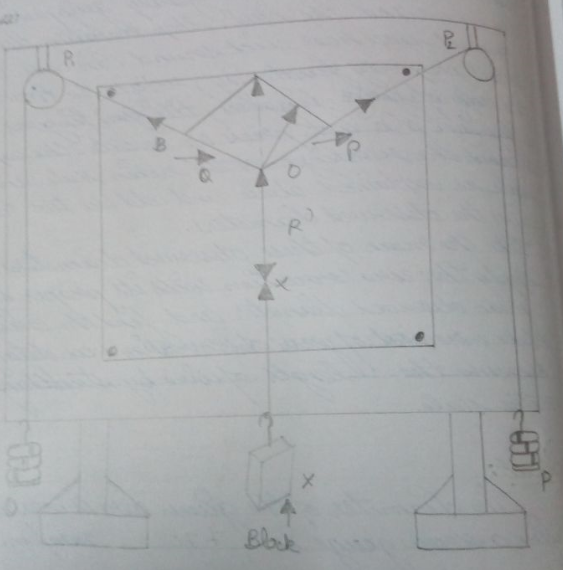## Result

– The unknown weight of given body is found to be 290 g.

## Viva Questions:-

______ represents the resultant of the vector addition in parallelogram law of vector.

Diagonal of the parallelogram

Friction in the pulleys is one of the sources of error in the experiment using Gravesand’s apparatus. True or False.

False

Mass is a vector quantity. True or False.

False

Define Vector

Physical quantities, having both magnitude and direction are called vector quantities.

Grab other Class XI Physics practicals from here.

## To measure the diameter and volume of a given wire using a screw gauge

Grab other Class XI Physics practicals from here.

## Apparatus:

Screw gauge, a thin wire and a metre scale

## Theory:

### Formulae used:

Where MSR = Main scale reading
and, CSR = nxLC
Where n = no of circular scale division coinciding with main scale
LC = Least count of screw gauge
Therefore, total reading = MSR = (nxLC)

ii) If DD be the mean diameter and l be the length of the wire, then volume of wire
V = π(D/2)2l = (πD2l)/4

## Procedure:

### Measurement of the Diameter

1. First of all calculate the pitch and the least count of the given screw gauge.
2. Find the zero error with its proper sign. Even after when the zero error is nil, this fact too should be recorded.
3. Now insert the wire between the screw and the steed of the screw gauge. Move the screw forward by rotating the ratchet till the wire is gently gripped between the screw and the steed. Stop rotating the ratchet the moment you hear click sound. In case the ratchet is not properly functioning, rotate the circular cap with the help of your fingers, till your fingers start slipping. Do not press or rotate the cap too hard. Record the readings on the main scale and the circular scale as explained above and add the two readings. This gives the observed diameters.
4. Take the mean of these observed diameters.
5. Apply the zero correction with its proper sign to the mean observed diameter and find the correct diameter.
6. Make a record of your observations as detailed below.
7. Measure the length of wire by stretching it along a metre scale.

## Sources of Error:-

• Backlash error: It occurs due to wear and tear of the screw threads, it is observed that reversing the direction of rotation of the thimble, the tip of the screw does not start moving in the opposite direction immediately, but remains stationary for a part of rotation. This is called back lash error. It occurs if we move the screw in one direction and then in opposite directions repeatedly.
• Zero error: If on bringing the flat end of the screw in contact with the stud, the zero mark of the circular scale coincides with the zero mark on base line of the main scale, the instrument is said to be free from zero error. Otherwise an error is said to be there.

## Observations

:-

 Main/Linear Scale Reading (M.S.R.) Circular Scale Reading (C.S.R.) Total observed reading (D) = M.S.R. + (nxLC) (mm) No of circular scale division in line with main scale (n) n x L.C.(mm) 8 43 0.043 8.043 8 47 0.047 8.047 8 46 0.046 8.046

Length of the wire = 27cm

## Calculations

:-

Mean Observed Reading = (8.043+8.047+8.046)/3 = 8.0453 mm

Volume of wire = (πD2l)/4 = π(8.0453/1000)2(0.27)/4 = 0.00001372757m3

## Diagram

:-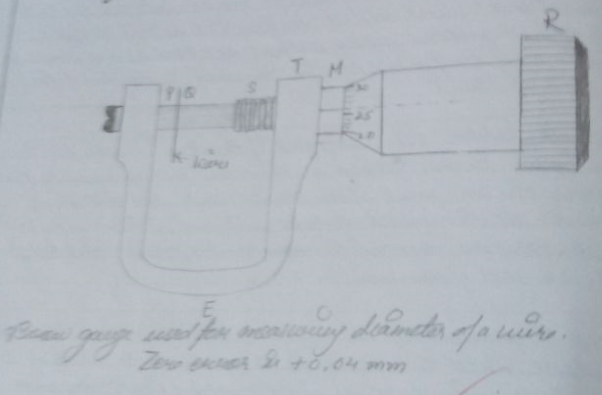Result – The diameter of the given wire is measured by using a screw gauge is 8.0453 mm. The volume of the wire is 0.00001372757m3.

## Viva Questions:-

What is least count?

The distance through which the screw advances when it is rotated through one division of the head scale.

What is the least count of the screw gauge if the head scale of a screw gauge contains 100 divisions and its pitch is 1 mm?

0.01mm

Screw gauge is less reliable than Vernier calliper in measuring the dimensions of an object. True or False.

False

What is screw gauge commonly referred to as?

Micrometer

What is ratchet’s function in a screw gauge?

Ratchet prevents screw gauge from undue tightening.

Grab other Class XI Physics practicals from here.

## Drawing I-V characteristics graph of p-n junction diode in forward biasing and to find state and dynamic resistance.

You can also get Class XII Practicals on BiologyPhysics, and Physical Education.

Aim : To draw I-V characteristics graph of p-n junction diode in forward biasing and to find state and dynamic resistance.

Apparatus : A p-n junction (semi conductor) diode, a 3 volt battery, a 50 volt battery, a high resistance rheostat, one 0-3 volt voltmeter, one 0-50 volt voltmeter, one 0-100 mA ammeter, a 0-100 uA ammeter, one way key, connecting wires & sand paper.

Theory :

When the p section of the diode is connected to positive terminal and of a battery and n section is connected to negative terminal of the battery then junction is said to be forward bias with an increase in bias voltage the forward current increases slowly in the beginning and then rapidly. At about 0.7 V for SI diode (0.1 V for Ge), the current increase suddenly. The value of forward bias voltage, at which the forward current increases rapidly, is called cut in voltage or threshold voltage.

Procedure

• Make circuit as shown in the circuit diagram.
• Make all the connection neat and tight.
• Note at least count and zero error of voltmeter (V) and milliammeter (mA)
• Bring moving contact of the potential divider (rheostat) near the negative end. Insert the key K. Voltmeter V and milliammeter mA will give zero reading.
• Move the contact a little towards the positive end to apply a forward bias voltage (vF) of 0.1 V current remains zero.
• Increase the forward bias voltage up to 0.3 V for Ge diode.
• Current remains zero ( it is due to junction potential barrier of 0.3 V)
• Increase VF to 0.4 V Milli ammeter records a small current.
• Increase Vf in steps to 0.2 V and note the corresponding current. Current increase first slowly and then rapidly till Vf becomes 0.7 V.
• Make VE = 0.72 V. The current increases suddenly. This represents “ forward breakdown” stage.
• If the VF increases beyond the “ forward breakdown” stage the forward current doesn’t change much. Now take out the key at once.

Result

This junction resistance for forward bias :

Resistance (static ) = 28.5 ohm.

Dynamic resistance = 0.02 ohm.

Precaution

• All connection should be neat and tight.
• Key should be used in circuit and opened when not in use.
• Forward bias voltage beyond breakdown shouldn’t be applied.

Sources of error

The junction diode applied may be faulty.

Circuit diagram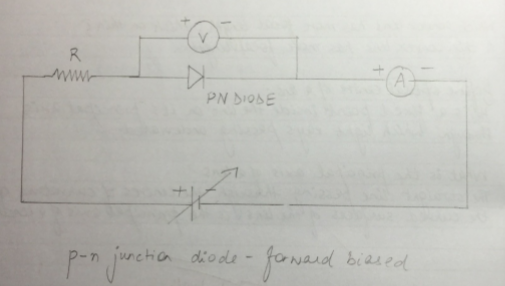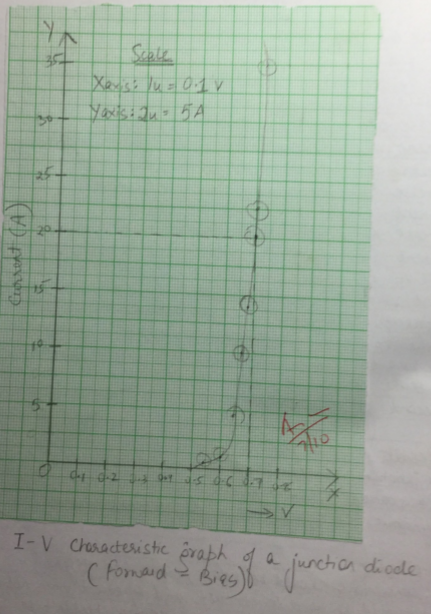Observation Table

 S No Potential diff across diode (v) Current (A) 1 0.1 0 2 0.2 0 3 0.3 0 4 0.4 0 5 0.5 0 6 0.54 1 7 0.6 2 8 0.62 4 9 0.64 5 10 0.66 10 11 0.68 13 12 0.7 20 13 0.7 25 14 0.72 30 15 0.72 35

Calculation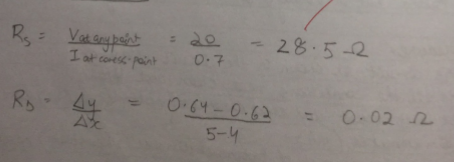Viva question

What is a function ?

It is a common surface of n type and p type semiconductor.

What is junction potential barrier ?

The potential difference between junction ends of the two types of semi conductor is called junction potential barrier.

What are the two types of biasing ?

1 forward biasing 2 Reverse biasing

Why is a forward bias so called ? How does it affect junction resistance ?

Forward bias so called because it makes free charge carrier to move forward towards junction . It increases the junction resistance (make less).

You can also get Class XII Practicals on BiologyPhysics, and Physical Education.

## Converting the given galvanometer into a voltmeter of desired range and to verify the same

You can also get Class XII Practicals on BiologyPhysics, and Physical Education.

Aim : To convert the given galvanometer into a voltmeter of desired range and to verify the same .

Apparatus : A Weston type galvanometer , a voltmeter of 0-3 V range , a battery eliminator , two resistance boxes , the one way key , a rheostat , connecting wires .

Theory : the series resistance required for conversion ,

R=V/Ig-G

Where V is the range of conversion .

Procedure :

1. Calculate the value of series resistance R to be connected In series with the galvanometer for the given V .
2. Connect a resistance box in series with the galvanometer and take out the plug of R . The galvanometer is now ready for use as voltmeter of range V volts .
3. For verification , make the connection as shown in diagram . A and B are the fixed terminals and C is the variables terminals .
4. Take out the plugs of calculated R from the resistance box . Insert a key K and adjust the movable contact of the rheostat so that deflection in the galvanometer becomes maximum .
5. Note the readings of voltmeter and galvanometer . Convert the galvanometer reading into volts.
6. Find the difference , if any between the readings of voltmeter and galvanometer . The difference gives the error .
7. Move the variable contact C and take five observation covering whole range of voltmeter .

Result

As the difference in actual and measured value of potential difference is very small , the conversion is perfect .

Precaution

1. Length of the shunt wire should be neither too short nor too long .

2. The voltmeter used for verification should preferable be of the same range as the range of conversion .

3. Value of required series resistance should be calculated accordingly .

Sources of error

The connection may be loose .

The emf of the cell or battery may be variable .

Circuit diagram :-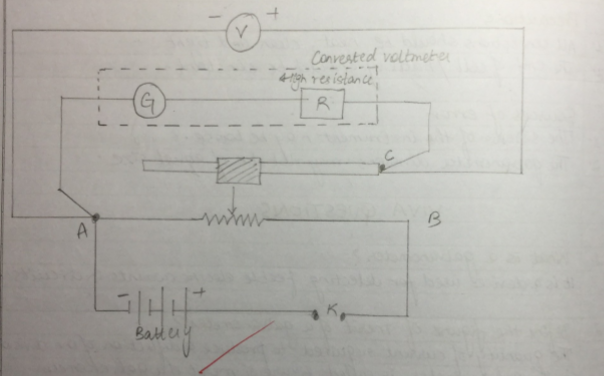Observation

Resistance of given galvanometer (G) 60 ohm

No of division (n)=10

Current (Jg) =nk=0.00028A

Range of conversion = V

=0-3 V

Resistance to be placed in series = Rs

Rs =V/Ig-G

Rs =3/0.00028 -60.7

Rs = 10653.5 Ohm

Verification

V1 =3V

V2=2.8 V

% error = 3-2.8/3 x 100

=0.2/3 x 100

=6.67 %

Viva question

Why is a Galvanometer not suitable to work as an ammeter .

A galvanometer has more resistance and less current . carrying capacity from those required in ammeter . It will damage if large current flows through it .

What is a shunt ?

A small resistance connected in parallel with a galvanometer is called shunt .

Why is a galvanometer not suitable to work as voltmeter ?

A galvanometer has less resistance and more current carrying capacity from those required by a voltmeter .

How is a galvanometer converted into a voltmeter ?

A galvanometer is converted into a voltmeter by connecting a high resistance in series with the galvanometer coil.

What happens when a voltmeter is converted in series in a circuit ?

The voltmeter cannot measure the potential difference in the circuit because overall resistance of circuit increases .

You can also get Class XII Practicals on BiologyPhysics, and Physical Education.

## Finding the focal length of a convex lens by plotting graph between u and v

You can also get Class XII Practicals on BiologyPhysics, and Physical Education.

Aim : To find the focal length of a convex lens by plotting graph between u and v.

Apparatus : An optical bench with three upright (central upright fixed , two outer uprights with lateral movements ), a convex lens with lens holder , two optical needle , (one thin , one thick ) a knitting needle and a half meter scale .

Theory : The relation between u , v and for a convex lens is

1/f= 1/v – 1/u Where f=focal length of convex lens

i/f = u-v/uv u =distance of object needle from optical center of the lens (-)

f =uv/u-v v = distance of image needle from optical from optical center of lens (+)

Procedure

1. Mount the concave mirror in the mirror holder .
2. Obtain the image of a tree or building outside of the window on a screen and move the mirror forward and backwards to get a sharp image on the screen .
3. Measure the distance between mirror and screen (rough focal length ).
4. Clamp the holder with lens fixed upright at 50cm mark .
5. Adjust the lens so that its surface is vertical and upright keep it fixed .
6. Take the thin optical needle as object needle (o).Mount it in outer laterally moveable upright near zero end .
7. Move the object needle upright and clamp it at a distance nearly 1.5 times the rough focal length obtained earlier .
8. Adjust height of the object needle to move its tip lie on a horizontal line through the optical center of the lens .
9. Note the position of the index mark on the base of object needle .
10. With the eye closed , see with the right eye from the other end of the optical bench . An inverted and enlarged image of the object needle will be seen .
11. Mount the thick optical needle in the fourth upright near the other end of the optical bench .
12. Adjust the height of the image needle (thick) so that its tip is seen in line with the tip of the image when seen with the right eye open .
13. Move the eye towards right .The tip will get separated .The image tip and the image needle tip have parallax .
14. Remove the parallax tip to tip .
15. Note the position of the index mark on base of image needle .
16. Record the position of the index mark on the base of upright of lens , the object and image needle .
17. Find index correction (if any ) as described for distance between optical center of lens and tip of image or object needle .
18. Move object needle upright towards mirror in steps of 1 cm to get observation .Repeat the experiment .
19. Move object needle upright towards mirror in steps of 1 cm to get the rest of the observation . Repeat the experiment .

Result

F =uv /u-v f from u-v graph

Diagram

Focal length of convex lens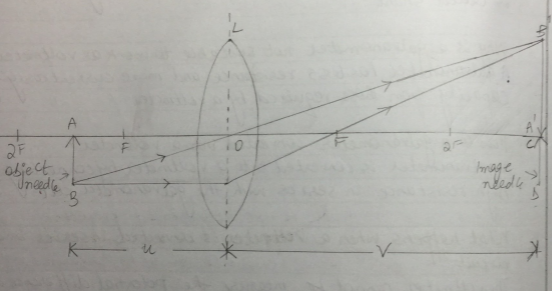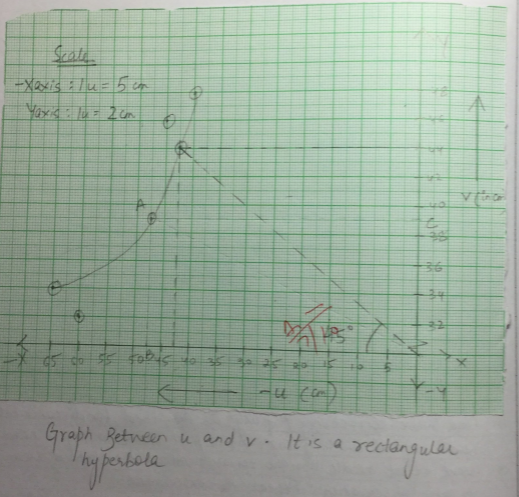Observation table

 S No Position of lens (cm) Object length u (inch) Image lens v (in cm) Position of object Position of image 1/u 1/v 1 80 60 32 20 11.2 0.0107 0.0312 2 80 65 34 25 11.6 0.018 0.29 3 80 50 39.3 30 121.3 0.02 0.054 4 80 45 46 35 126 0.122 0.204 5 80 40 48 40 135 0.25 0.181

Total = 0.312 cm 0.0167cm

Calculation

1/f=1/v-1/u

=0.0312 –(0.0167)

0.0479cm

F =1/0.479

Precaution

1. Tip of the object and image needle should lie at same height as the centre of the lens .
2. Parallax should be removed from tip to tip.

Sources of error

1. The upright may not be vertical .
2. Parallax removed may not be perfect .

Viva questions

Define and give lens formula .

The equation relating the object distance (u) , the image distance (v) and lens focal length (f) is called lens formula 1/u+ 1/v = 1/f.

What are the various assumption made in deriving lens formula ?

The following assumption are made :

The lens is thin .

The lens has a small .

The point object lies on to the principal axis and placed perpendicular

The incident ray make small angles with the lens surface on the principal axis .

Which convex lens has more focal length , thick or thin ?

A convex lens has more focal length .

Define optical centre of a lens ?

It is a fixed point inside the lens on its principal axis through which light ray passing undeviated .

What is the principal axis of a lens ?

The straight line passing through the centre of curvature of the curved surfaces of the lens is the principal axis of a lens .

You can also get Class XII Practicals on BiologyPhysics, and Physical Education.

## Determining the internal resistance of a given primary cell using cell using potentiometer

Aim : To determine the internal resistance of a given primary cell using cell using potentiometer .

Apparatus : a potentiometer , a battery , (or eliminator ) , two one way key , a rheostat of low resistance , a galvanometer , a high resistance box , a fractional resistance box , an ammeter , a voltmeter , a cell , a jockey , a set square , connecting wires , a piece of sand paper .

Theory

When Key K2 is open and K1 is closed ,

Let null point be obtained at a distance l1 from A

: E =Kl1 (1)

When key K2 is closed and K1 is open ,

Let null point be obtained at a distance l2 from A

V=Kl2 (2)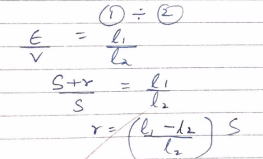Where S is the shunt resistance in parallel with given cell .

l1 and l2 ; balancing length without & with shunt respectively .

r: internal resistance of the cell.

Procedure

Make the connection as shown in diagram .

Clean the ends of the connecting wires with sand paper and make tight connection , tighten the plug of the resistance box .

Check the emf of the battery and cell and see the emf of the battery is more than that of the given cell other wise null or balance point wont be obtained (E` >E).

Take maximum current from battery , making rheostat resistance small.

Insert the plug key k , and adjust the rheostat so that a null point is obtained on the fourth wire of the potentiometer .

Insert the 2000 ohm plug in its position in resistance box and obtain a null point by slightly adjusting the jockey .

Measure the balancing length l1.

Take out the 2000 ohm plug from the resistance box . introduce the plug in the key k1 as well in key k2 .Take out a small resistance from the resistance box R connected in parallel with cell.

Slide the jockey along the potentiometer wire and obtain a null point

Insert the 200 ohm plug back in its position in RB and make further adjustment for sharp null point .

Measure the balancing length l2 from end P.

Remove the plugs key k1 and K2 . wait for some time and repeat the activity for the same current .

Circuit Diagram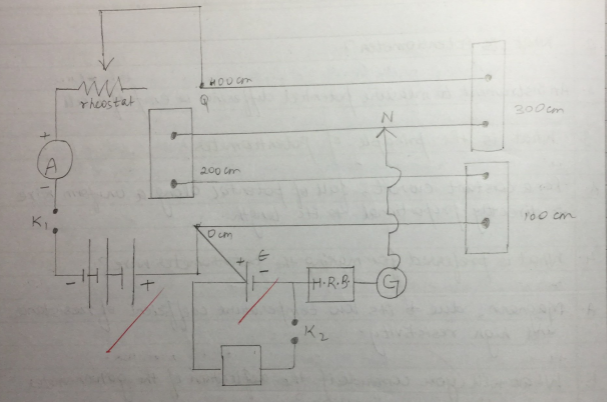Observation

 Value of Shunt resistance (S in ohm) Balance Length l1 (K2 is open) without Shunt (cm) Balance length l2 with Stunt (K2 is closed) (cm) r = [(l1-l2)S]/l2 (in ohm) Mean ‘r’ 1.5 171.4 64 1.67 1.77 ohm 2 171.3 61.5 1.78 2.5 171.1 59.6 1.87

Calculation

Mean ‘r’ = (1.67+1.78+1.87)/3 = 1.77 ohm

Result

The internal resistance (R) of given cell is 1.77 OHM

Precaution

For one set of observation the ammeter reading should be constant .

Current should be passed for short time .

Jockey should be rubbed against potentiometer wire .

Sources of error

The emf of the battery is less then the cell (E`<E)

Cell is disturbed during the experiment .

Viva Questions

What do you mean by the internal resistance of cell ?

It is the resistance offered by electrodes

What are the factors on which internal resistance of a cell depends ?

1 Nature of electrodes

2 Nature of electrolyte

3 concentration of electrolyte

4 Temperature of electrolyte

5 Distance between electrodes

6 Area immersed

Does the internal resistance depends on current drawn from the cell ?

Yes the internal resistance usually increases as more current is drawn fom the cell

Can we find the internal resistance of a secondary cell ?

No the internal resistance of a secondary cell is so small (0.01 ohm ) that this method can’t be used .

What should a cell not be disturbed during the experiment ?

Disturbing the cell may change the factors on which internal resistance depends

You can also get Class XII Practicals on BiologyPhysics, and Physical Education.

## Determining resistance of a galvanometer by half deflection method and to find its figure of merit .

Aim : To determine resistance of a galvanometer by half deflection method and to find its figure of merit .

Apparatus : A Weston type of galvanometer , a voltmeter , a battery /battery eliminator , two 10,000 ohm and 200 ohm ) resistance boxes , two one way key , a rheostat , a screw gauge , a meter scale , an ammeter of given range , connecting wires and a piece of sand paper .

Theory

The resistance of the given galvanometer as found by half deflection method .

G= (RxS)/(R-S) (1)

Where R is the resistance connected in series with the galvanometer and S is the shunt resistance .

The figure of merit , K =E /[(R+G)Q] (2)

Where E is the end of the cell and Q is the deflection produced with resistance R.

The maximum current that can pass through the galvanometer , Ig=nk

Where n is the total number of dimension on the galvanometer scale on either side of zero .

Procedure

1. Resistance of galvanometer by half deflection method

• Make the connections accordingly as shown in circuit diagram .
• See that all plugs of the resistance boxes are tight
• Take out the high resistance (200 Ohm ) from the resistance box R and insert key K1 only .
• Adjust the value of R1 so that deflection is maximum , even in number and within the scale .
• Note the deflection . Let it be Q.
• Insert the key k2 also and without changing the value of R2 ,adjust the value of S , such that deflection in the galvanometer reduces to exactly half the value of resistance S.
• Note the value of resistance S .
• Repeat step 4 & 7 three times taking different R and adjusting .

2. Figure of merit

• Take one cell of the battery and find its emf by a voltmeter by connecting +ve of the voltmeter with +ve of cell and – ve of the voltmeter with -ve of cell . Let it be E.
• Take connection as in circuit diagram .
• Adjust the value of R to obtain a certain deflection Q when circuit is closed .
• Note the value of resistance R and deflection Q.
• Now change the value of R and note the galvanometer deflection again .
• Repeat the step with both cells of the battery with different voltages like 2,4,6 ,8 volts from battery eliminator.
• Find the figure of merit K using the formula

Observation table

 Resistance (R) Deflection (Q) Shunt (S) G =(RxS)/(R-S) 5000 10 5 5.005 10000 6 3 3 2000 20 10 10.05 1500 26 3 13.11 Average G = 7.79 ohms
 Resistance (R) Deflection (Q) K 5000 9 0.556 10000 5 0.60 2000 20 0.502 1500 25 0.524 Average K = 0.5455 A/dn

Calculations

1. G = (5.005+3+10.05+13.11)/4 [E = 1.45V]
2. Figure of Merit : Current in the galvanometer per unit time
3. K = IQ = [(E)/(R+G)]x[1/Q] – SI unit = AD division = 10-5 Al div

Result

Resistance of given galvanometer is 7.79 ohm .

Figure of merit of given galvanometer is 0.545 A/dn .

Precautions

All connections should be neat and tight .

The emf of cell / battery should be constant .

Source of error

The scene of the instrument may be loose .

The galvanometer division may not be of equal size.

Diagram

Resistance of Galvanometer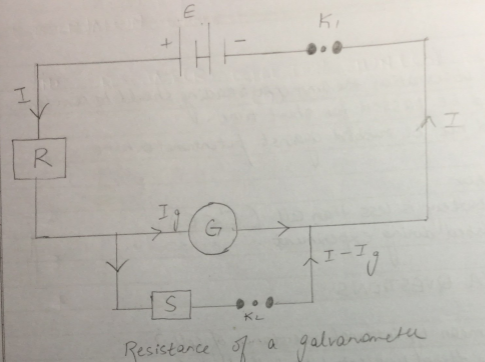Figure of Merit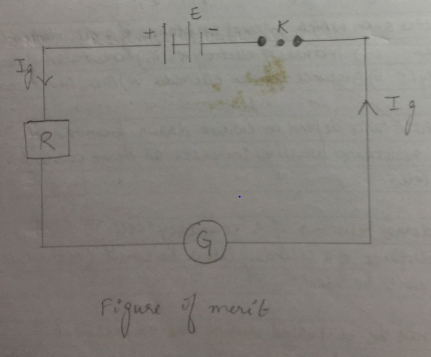Viva question

What is the galvanometer ?

It is a device used for detecting feeble electric currents in circuit .

Define the figure of merit of a galvanometer ?

The quantity of current required to produce a deflection of one division in the galvanometer is called figure of merit of a galvanometer . It is represented by K . Its unit is ampere per division .

Why is this method called half deflection method ?

It is so because the deflection is made half by using a shunt resistance S.

Under what condition id the resistance of a galvanometer (E1) equal to shunt resistance (S)?

E1=S , only when series resistance R is very high .

You can also get Class XII Practicals on BiologyPhysics, and Physical Education.

## To compare EMF of two given primary cells using potentiometer

Aim : To compare EMF of two given primary cells using potentiometer

Apparatus : potentiometer , a leclanche cell , a daniel cell , an ammeter , a voltmeter , a galvanometer , a battery , (battery eleminator), a rheostat , of low resistance , a resistance box , a one way key , a two way key , a jockey , a set square , connecting wire , a piece of sand paper .

Theory

When we keep key (K1) closed and (K2) open , let the null point found be lAJ1

E1=KlAj1 (1)

When we keep K1 open and K2 closed , let null point obtained by lAJ2 .

E2=KlAj2 (2)

(1)/ (2)

E1 / E2 = KlAJ1 / KlAj2

E1/E2 =l1/l2

Where E1 and E2 are the emf of two given cells .

Procedure

1. Arrange the apparatus as shown in the circuit diagram .
2. Connect the positive poles of the cells to the terminal and the negative poles to the terminal a and b of the two way key .
3. Insert the plug in the key K and also in between the terminals a and c of the two way key .
4. Slide the jockey gently over the potentiometer wires until you obtain a point of no deflection .
5. Note the length l1 at the point.
6. Repeat this with E2 by disconnecting E1 and inserting plug into gap a and c of two way key .
7. Record l2 at null point .
8. Repeat this different resistance .

Observation table

 Balancing Lengths E1 / E2 = lAJ1 / lAj2 L1 for cell E1 (cm) L2 for cell E2 (cm) 327 376 0.86 323.5 371 0.87 321.5 369 0.87 312.5 352.5 0.88

Result

The ratio of emf E1/E2 ~0.87

Precaution

1. The connections should be neat, clean and tight.
2. The plugs should be introduced in the keys only when the observations taken.
3. The positive poles of the battery E and cells Ex and E2 should, all be connected to terminal at the zero of the wires.
4. The jockey key should not be rubbed along the wire. It should touch the
5. The e.m.f. of the battery should be greater than the e.m.f.’s of the either cells.
6. Some high resistance plug should always be taken out from resistant the jockey is moved along the wire.

Sources of error

1. The auxiliary battery may not be fully charged.
2. The potentiometer wire may not be of uniform cross-section and material. throughout its length.
3. End resistances may not be zero.

Diagram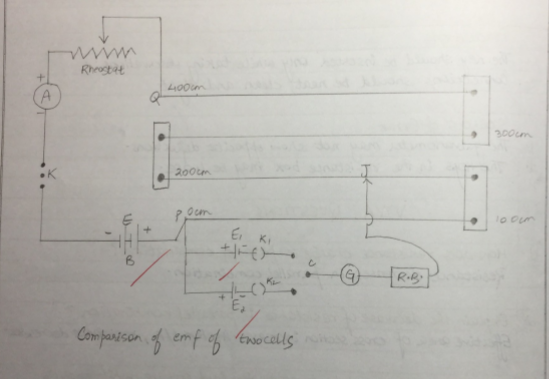VIVA Question

What is emf of a cell?

Electromotive force of a cell is the potential difference across the terminals of the cells when it is in open circuit i.e. no current is drawn from the circuit .

What is potentiometer ?

An instrument to measure potentiometer difference or emf of a cell .

What is the principle of potentiometer ?

For a constant current , fall of potentiometer along a uniform wire is directly proportional to its length .

What is preferred for making potentiometer wire ?

Magnum , due to its low temperature coefficient of resistance and high resistivity .

What will you conclude if the deflection of the galvanometer is in the same direction ?

The positive terminals aren’t connected at one point

Potential difference between wires ends is less than emf of cells to be measured .

Emf of driving cells is less than that of cells to be measured .

You can also get Class XII Practicals on BiologyPhysics, and Physical Education.

## To verify the laws of combination (series) of resistance using a meter bridge (R=R1 + R2 )

Aim : To verify the laws of combination (series) of resistance using a meter bridge (R=R1 + R2 )

Apparatus : a meter bridge , a leclanche cell (battery eliminator ) , a galvanometer , a resistance box , a jockey , two resistance wire or two resistance coils , set square , connecting wires .

Theory

1. The resistance (r) of a wire or coil is given by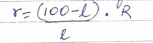Where R is the resistance from resistance box in the left gap and l is the length of the meter bridge wire from zero end upto balance point .

2. When two resistance r1 & r2 are connected in series , then their combined resistance

R3=R1+R2

Procedure

1. Mark the two resistance coil as r1 and r2 .
2. To find the r1 and r2 proceed same way as in experiment 1.

(if r1 and r2 are not known )

1. Connect the two coils r1 and r2 in series as shown circuit diagram in the right gap of meter bridge and find the resistance of this combination. Take at least three sets of observation.

Result

Within limits of experimental error , experimental and theoretical values of R  are same . Hence law of resistance in series is verified .

Precaution

1. The key should be inserted only while taking observation .
2. Connections should be neat and tight .

Sources of error

1. The key in resistance box may be loose .
2. The galvanometer may not show opposite deflection .

Circuit diagram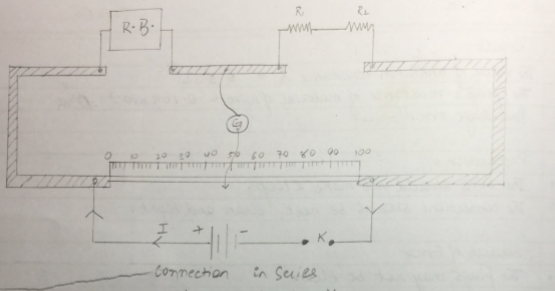Observation table

 Resistance in R.B. (ohm) Balance l (cm) (100-l) (cm) [(100-l)*R]/l Mean r1 (cm) 0.5 24 76 1.583 1.616 1 38 62 1.631 2 55 45 1.636
 Resistance in R.B. (ohm) Balance l (cm) (100-l) (cm) [(100-l)*R]/l Mean r2 (cm) 0.5 33 67 1.015 1.015 1 50 50 1 2 66 34 1.030
 Resistance in R.B. (ohm) Balance l (cm) (100-l) (cm) [(100-l)*R]/l Mean rs (cm) 0.5 16 84 2.625 2.615 1 28 72 2.571 2 43 57 2.651

Calculation

R1 + R2 = 1.616+1.015 = 2.631 ohm
Rs = 2.615 ohm

Therefore, experimental error = [(2.615-2.631)/2.615]*100 = 0.6%

Viva question

How does resistance change in series combination ?

Ans. Resistance increase in series combination

Why does resistance increase in series combination ?

Ans. Effective length of resistor increases . As R*l , resistance increases .

What is resistance ?

Ans. it is the opposition offered by the material of two wire to flow of electric current .

What is the cause of resistance ?

Ans. it is the opposition offered by the material of wire to flow of electric current .

What is the effect of temperature In the resistance of conductor ?

Resistance of all conductor increases with increase in temperature .

You can also get Class XII Practicals on BiologyPhysics, and Physical Education.

## Verifying laws of combination (parallel) of resistance using meter bridge

Aim: To verify laws of combination (parallel) of resistance using meter bridge .

Apparatus: a meter bridge , a leclanche cell , a galvanometer , a jockey , a jockey , a resistance box , two resistance wire , connecting wires , set square .

Theory

The resistance (r) of a resistance wire or coil is given by r=(100-l)/L *R where R is the resistance from resistance box in the left gap and L is the length of meter bridge wire from O end upto balance point.

When r1 and r2 are connected in parallel , then their combined resistance

Rp = r1r2 /r1+r2

Procedure

1. Mark the two resistance wires as r1 and r2
2. Connect the two coils r1 and r2 in parallel as shown in fig. in the right gap of meter bridge and find the resistance of this combination . Take at least three set of observation

Result

Within limit of experimental error , experimental and theoretical values of Rp are same .

Hence law of resistance in parallel are verified .

Precaution

The key should be inserted only while taking observation .

Connection should be neat and tight .

Source of error

The galvanometer may not show opposite deflection

The key in the resistance box may be close .

OBSERVATION TABLE

Value of r1

 Resistance of R.B. (ohm) Balance L (cm) (100-l) (cm) [(100-l)*R]/L Mean r1 0.5 59 41 0.347 0.6393 1 37 63 0.587 2 33 67 0.985

Value of r2

 Resistance of R.B. (ohm) Balance L (cm) (100-l) (cm) [(100-l)*R]/L Mean r2 0.5 83.2 16.8 0.1009 1.729 1 80.8 19.2 4.208 2 69.4 30.6 0.881

Value of rp

 Resistance of R.B. (ohm) Balance L (cm) (100-l) (cm) [(100-l)*R]/L Mean rp 0.1 27 73 0.27 0.36 0.2 32 68 0.42 0.5 56 44 0.39

Calculations

Rp = (R1R2)/R1+R2 = (0.693×1.729)/(0.693+1.729) = 0.4667 ohm

Experimental Error = [(0.36-0.4667)/(0.36)]*100 = 29.6%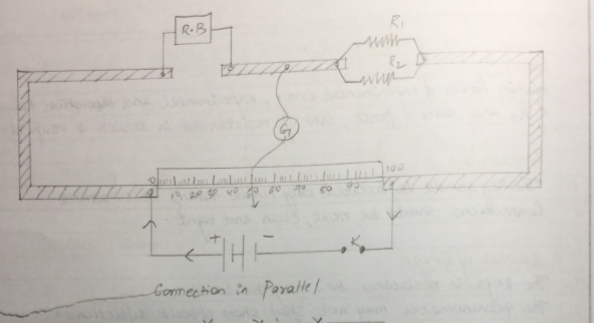Viva question

1. How does resistance change in parallel combination ?

Resistance decrease in parallel combination

2. explain the decrease of resistance in parallel combination ?

Effective area of cross section increases as r x 1/A , resistance decreases .

3. Why should jockey not be pressed too hard on wire while sliding ?

Sliding the jockey with a hard press will scratch the wire and make its thickness non-uniform .Resistance per unit length of wire will be inconsistent as resistance depends on area of cross section .

4. What is the resistance of an open key ? Explain.

Open key has infinite resistance as it makes current zero (R=V/I)

5. Define unit of resistance .

SI unit of resistance is OHM or volt per ampere . 1 Ohm is the resistance of a conduction carrying 1 ampere current when potential difference maintained across its end is 1 volt .

You can also get Class XII Practicals on BiologyPhysics, and Physical Education.